# Angular Interpolation

## Angular Interpolation with Examples

In this article, I am going to discuss the Angular Interpolation with Examples. Please read our previous article before proceeding to this article where we discussed the basics of Angular Data Binding. As we already discussed in our previous article, Interpolation Data Binding is used to achieve one way data-binding i.e. From Component to the View Template.

##### What is Angular Interpolation?

If you want to display the read-only data on a view template (i.e. From Component to the View Template), then you can use the one-way data binding technique i.e. the Angular interpolation.

The Interpolation in Angular allows you to place the component property name in the view template, enclosed in double curly braces i.e. {{propertyName}}.  So, the Angular Interpolation is a technique that allows the user to bind a value to a UI element.

##### Understanding Interpolation in Angular Application:

Let us understand how to use the Interpolation with some examples.

###### Step1: Modify the index.html

Please modify the index.html file as shown below.

```<!doctype html>
<html lang="en">
<meta charset="utf-8">
<title>MyAngularApp</title>
<base href="/">
<meta name="viewport" content="width=device-width, initial-scale=1">
<body>

<app-root></app-root>

</body>
</html>
```
##### Step2: modify app.component.ts file.

In order to understand Angular Interpolation binding, please have a look at the following image. Here, we have created two string variables (FirstName and LastName) within the component (i.e. AppComponent) and initialize these two variables with some default values. Then please focus on the template property of the @Component decorator. Here, the angular framework gets the value of the FirstName and LastName property from the component (i.e. AppComponent) and then inserts the FirstName and LastName value between the opening and closing <h1> element. Here, we are using double curly braces to place to component properties and this is called Interpolation binding in Angular Application.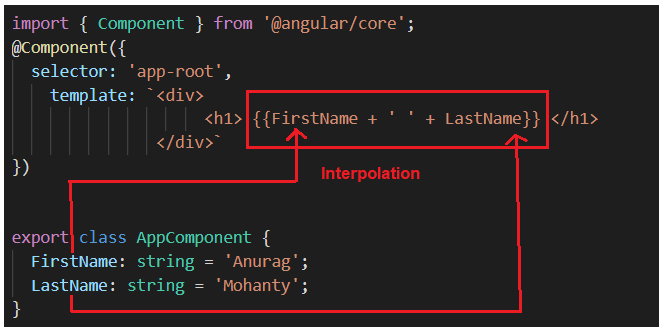So, open app.component.ts file and then copy and paste the following code in it.

```import { Component } from '@angular/core';
@Component({
selector: 'app-root',
template: `<div>
<h1> {{FirstName + ' ' + LastName}} </h1>
</div>`
})

export class AppComponent {
FirstName: string = 'Anurag';
LastName: string = 'Mohanty';
}
```

And, when you run the application, then it should display the FirstName and LastName in the browser as shown in the below image.##### Angular Interpolation with hardcoded string:

It is also possible in Angular to concatenate some hard-coded string value with the property value. In order to understand this, let us add the hard-coded string First Name before the FirstName Property and Last Name before the LastName property. If this is your requirement then you could do this as shown in the below image.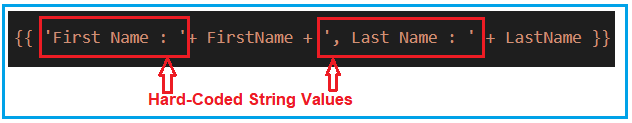So, please modify the app.component.ts file as shown below.

```import { Component } from '@angular/core';
@Component({
selector: 'app-root',
template: `<div>
<h1> {{ 'First Name : '+ FirstName + ', Last Name : ' + LastName }} </h1>
</div>`
})

export class AppComponent {
FirstName: string = 'Anurag';
LastName: string = 'Mohanty';
}
```

With the above changes in place, now you should get the FirstName and LastName property values along with the hardcoded string values as shown in the image below.##### Angular Interpolation with Expression:

In Angular using Interpolation (i.e. double curly braces), you can also specify some valid expression. For example, if you want to perform mathematical calculations then you can do such calculations using interpolation as shown in the below image.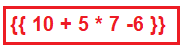So, modify the app.component.ts file as shown below and then see the output in the browser.

```import { Component } from '@angular/core';
@Component({
selector: 'app-root',
template: `<div>
<h1> 10 + 5 * 7 - 6 = {{ 10 + 5 * 7 -6 }} </h1>
</div>`
})

export class AppComponent {
}
```
###### Output: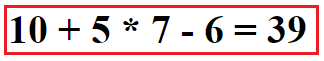It is also possible in Angular Interpolation to combine the expression with the property value. Let us understand this with an example. Please have a look at the following image. We have Salary property and we need to apply .10 as a bonus. So, here we can combine the expression with the property using Angular Interpolation.So, please modify the app.component.ts file as shown below and see the output.

```import { Component } from '@angular/core';
@Component({
selector: 'app-root',
template: `<div>
<h1> Bonus = {{Salary * .10 }} </h1>
</div>`
})

export class AppComponent {
Salary : number = 100000;
}
```

With the above changes in place, now it should display the following output.##### Interpolation in Angular with Ternary Operator:

The expression that is enclosed in the double curly braces is commonly called Template Expression and the template expression can also be a ternary operator. Please modify the app.component.ts file as shown below.

```import { Component } from '@angular/core';
@Component({
selector: 'app-root',
template: `<div>
<h1> Last Name : {{ LastName ? LastName : 'Not Available' }} </h1>
</div>`
})

export class AppComponent {
LastName : string = "Mohanty";
}
```

In the above example, the LastName property has a value i.e. Mohanty, So, you can see the last name property in the browser as shown in the below image.Now let’s modify the LastName property value to null as shown in the below code and then see the output in the browser.

```import { Component } from '@angular/core';
@Component({
selector: 'app-root',
template: `<div>
<h1> Last Name : {{ LastName ? LastName : 'Not Available' }} </h1>
</div>`
})

export class AppComponent {
LastName : string = null;
}
```

Now, when you run the application it will display the below output in the browser.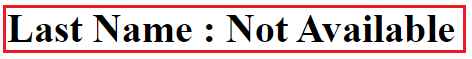##### Method Interpolation in Angular Application:

Let’s see how to create a method using typescript and then we will discuss how to call a class method using interpolation. So, what we will do here is, we will create one method let say GetFullName, and that method will return the full name by combining the first name and last name properties as shown in the below image. Here, you can access the class member using this operator.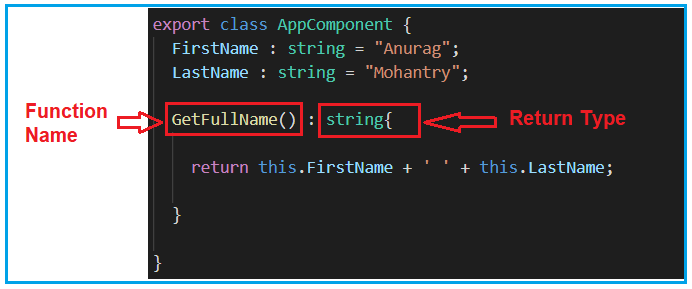Once you have a function created in the component, then you can call this function using the interpolation as shown below.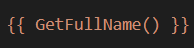So, modify the app.component.ts file as shown below and then see the output.

```import { Component } from '@angular/core';
@Component({
selector: 'app-root',
template: `<div>
<h1> Full Name : {{ GetFullName() }} </h1>
</div>`
})

export class AppComponent {
FirstName : string = "Anurag";
LastName : string = "Mohantry";

GetFullName() : string{
return this.FirstName + ' ' + this.LastName;
}
}
```

When you run the application, you should get the following output in the browser.##### Displaying Images using Angular Interpolation:

It is also possible in angular to display images using Interpolation. Let us say we want to display the image from the below link.

Modify the app.component.ts file as shown below to display images.

```import { Component } from '@angular/core';
@Component({
selector: 'app-root',
template: `<div>
<img src = {{ImagePath}} />
</div>`
})

export class AppComponent {
}
```

When you run the application, it will display the following image in the browser.1.2.3.